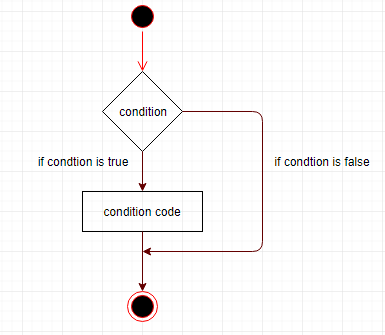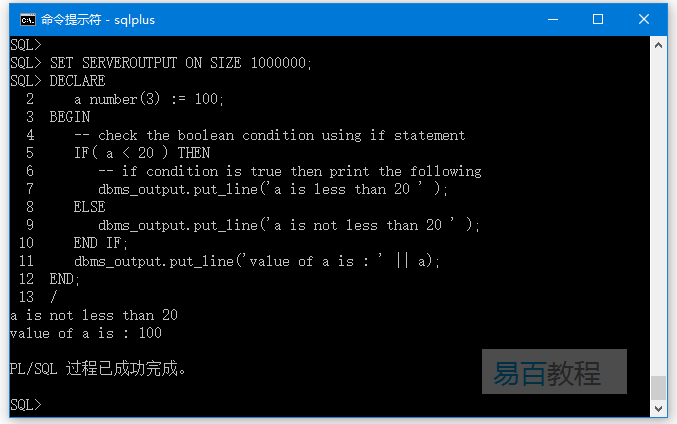IF-THEN语句的序列之后的ELSE语句的可选序列，ELSE语句块在IF条件为FALSE时执行。

IF-THEN-ELSE语句的语法是 -

IF condition THEN
S1;
ELSE
S2;
END IF;

IF color = red THEN
dbms_output.put_line('You have chosen a red car')
ELSE
END IF;## 示例

SET SERVEROUTPUT ON SIZE 1000000;
DECLARE
a number(3) := 100;
BEGIN
-- check the boolean condition using if statement
IF( a < 20 ) THEN
-- if condition is true then print the following
dbms_output.put_line('a is less than 20 ' );
ELSE
dbms_output.put_line('a is not less than 20 ' );
END IF;
dbms_output.put_line('value of a is : ' || a);
END;
/